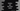# Learn to get the class name in Python in 2 ways## 2 different ways in Python to get the class name:

In this post, I will show you 2 different ways in Python to get and print the class name of an instance. This is commonly used in printing the class name in logs. You can create a common method for logging and call this method from different files or from different class instances. If you print the class name with other logs, it becomes easy to debug.

### Method 1: By using class.name:

We can use the class attribute of python. This attribute has one variable called name which is the name of the class.

class attribute can be accessed in an object of a class or in an instance of a class. Let’s take an example:

``````class Student:
def sayhello():
print('Hello from Student')

class Teacher:
def sayhello():
print('Hello from Teacher')

s = Student()
t = Teacher()

print(f'Class name of s is: {s.__class__.__name__}')
print(f'Class name of t is: {t.__class__.__name__}')``````

Here,

• We created two classes Student and Teacher.
• s is an object of the Student class and t is an object of the Teacher class.
• The last two print statements are printing the class names of these two objects.

If you run this program, it will print the below result:

``````Class name of s is: Student
Class name of t is: Teacher``````

If you call it on different objects of the same class, it will print the same result.

``````class Student:
def sayhello():
print('Hello from Student')

s = Student()
s1 = Student()
s2 = Student()

print(f'Class name of s is: {s.__class__.__name__}')
print(f'Class name of s1 is: {s1.__class__.__name__}')
print(f'Class name of s2 is: {s2.__class__.__name__}')``````

It will print:

``````Class name of s is: Student
Class name of s1 is: Student
Class name of s2 is: Student``````

### Method 2: By using type():

type() function returns the class type for a object. It takes one object as the parameter and returns the type of that object.

For example:

``````class Student:
def sayhello():
print('Hello from Student')

s = Student()

print(f'Class type of s is: {type(s)}')``````

It will print:

``Class type of s is: <class '__main__.Student'>``

We can use the name attribute of this value to get the class name. Let’s try it:

``````class Student:
def sayhello():
print('Hello from Student')

s = Student()

print(f'Class name of s is: {type(s).__name__}')``````

It will print:

``Class name of s is: Student``

Let’s try with multiple objects:

``````class Student:
def sayhello():
print('Hello from Student')

s = Student()
s1 = Student()
s2 = Student()

print(f'Class name of s is: {type(s).__name__}')
print(f'Class name of s1 is: {type(s1).__name__}')
print(f'Class name of s2 is: {type(s2).__name__}')``````

It will print the same output.